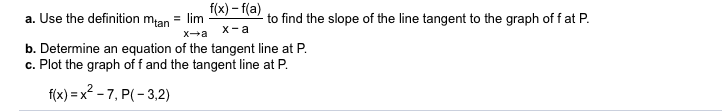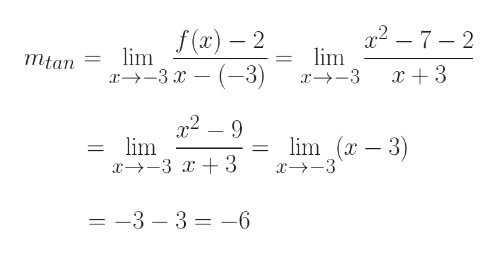# f(x)-f(a)a. Use the definition mtan= limto find the slope of the line tangent to the graph of f at Pх-аXab. Determine an equation of the tangent line at P.c. Plot the graph of f and the tangent line at Pf(x)=x2-7, P(-3,2)

Question
18 viewshelp_outlineImage Transcriptionclosef(x)-f(a) a. Use the definition mtan = lim to find the slope of the line tangent to the graph of f at P х-а Xa b. Determine an equation of the tangent line at P. c. Plot the graph of f and the tangent line at P f(x)=x2-7, P(-3,2) fullscreen
check_circle

Step 1

Part (a) P(-3, 2) = [a, f(a)]

Hence, a = -3, f(a) = 2

Please see the whiteboard for the calculation of mtan = slope of the tangent line = -6help_outlineImage TranscriptioncloseГа) — 2 x2- 7-2 lim x--3 lim 1—-3х — (—3) mtan 2-9 lim x 3 3 lim (x -3) =-3 - 3 -6 fullscreen
Step 2

Part(b)

Equation of the tangent line at P will be:

[y - f(a)] = mtan[x - (-3)]

Hence,...

### Want to see the full answer?

See Solution

#### Want to see this answer and more?

Solutions are written by subject experts who are available 24/7. Questions are typically answered within 1 hour.*

See Solution
*Response times may vary by subject and question.
Tagged in

### Functions